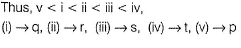Courses

# Test: Hydrogen Bonding

## 17 Questions MCQ Test Chemistry for JEE | Test: Hydrogen Bonding

Description
This mock test of Test: Hydrogen Bonding for JEE helps you for every JEE entrance exam. This contains 17 Multiple Choice Questions for JEE Test: Hydrogen Bonding (mcq) to study with solutions a complete question bank. The solved questions answers in this Test: Hydrogen Bonding quiz give you a good mix of easy questions and tough questions. JEE students definitely take this Test: Hydrogen Bonding exercise for a better result in the exam. You can find other Test: Hydrogen Bonding extra questions, long questions & short questions for JEE on EduRev as well by searching above.
QUESTION: 1

### Direction (Q. Nos. 1-12) This section contains 12 multiple choice questions. Each question has four choices (a), (b), (c) and (d), out of which ONLY ONE option is correct. Q. Which of the following compounds are soluble in H2O ?

Solution: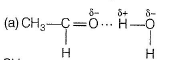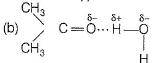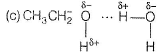All these are soluble in water due to intermolecular H-bonding.

QUESTION: 2

### Which of the following has maximum boiling point?

Solution:

Due to intermolecular H-bonding at two terminals boiling point of glycol is maximum.

QUESTION: 3

### In which of the following boiling point of Column I is not higher than that of Column II?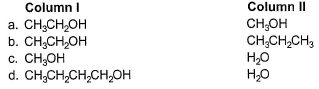Solution:

(a) Intermolecular H-bonding, boiling point increases with molecular weight for compounds within the same family.
(b) H-bonding is absent is CH3CH2CH3.
(c) Methanol is the larger molecule but H2O has more H-bonding because of two O—H bonds.
Thus, boiling point of H2O(ll) > CH3OH(l).
(d) Molecular weight of butanol is much higher and overcomes attractive forces of butanol.

QUESTION: 4

Which of the following correctly represents H-bonding?

Solution:

H-bonding is between polar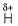and electronegative 0, N and F C-atom or H attached to C is not polar. Thus, (a), (c), (d) are incorrect.

QUESTION: 5

In dimer formation of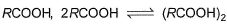which O-atom(s) is/are involved through H-bonding?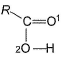Solution: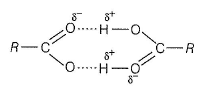QUESTION: 6

In the following compounds, select the points of interm olecular H-bonding (linear),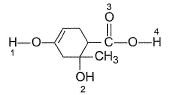Solution: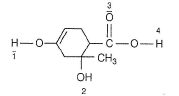1. Enoiic H, acidic and polar takes part in H-bonding.
2. 3° alcohol least acidic maximum basic.
3. Carbonyl oxygen is not involved.
4. Acidic and polar H, takes part in H-bonding.

QUESTION: 7

In the following alcohol, which — OH group is involved to the maximum extent in H-bonding?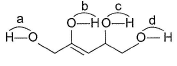Solution:

O — H group is attached to (ene) is m ost a cidic and thus, has maximum intermolecular H-bonding.

QUESTION: 8

Among the following sets, highest boiling points are of the species.

I. HF, HCI, HBr, HI
II. H2O, H2S, H2Se, H2Te
III. NH3,PH3, AsH3,SbH3

Solution:

F, O and N are electronegative elements. Thus, HF molecules are associated by intermolecules H-bonding giving it a liquid state and thus highest boiling point out of HF, HCl.HBr and HI.
Similarly, H2O has highest boiling point, and NH3 has highest boiling point.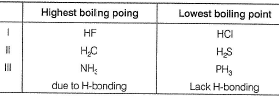QUESTION: 9

The H-bond is shortest in

Solution:

(EN) of S < 0 < F
Thus, H is attracted to larger extent by F atom making (H.......F) bond shortest.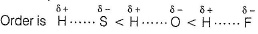QUESTION: 10

What is the dominant intermolecular force or bond that must be overcome in converting liquid CH3CH2OH to vapours?

Solution:

CH3CH2OH is in liquid state due to association as a result of intermolecular H-bonding.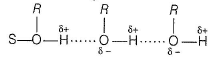QUESTION: 11

Number of water molecules directly attached to one water molecule is

Solution: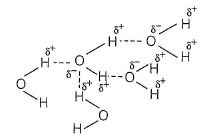One water molecule is directly attached to four water molecules. Thus, coordination number of water is 4.

QUESTION: 12

Consider the following compounds

I. HCI
II. HF
III. CH3COOH
IV. CH4
V. CH3OH
VI. CH3COO-

Q.
H-bonding is not present in

Solution:

HCI: EN of Cl is smaller, hence less polar than HF.
CH4 :non-polar
CH3COO- : H attached to C-atom is non-polar hence
HCI, CH4, CH3COO- — lack H-bonding.

*Multiple options can be correct
QUESTION: 13

Direction (Q. Nos. 13-16) This section contains 4 multiple choice questions. Each question has four choices (a), (b), (c) and (d), out of which ONE or  MORE THANT ONE  is correct.

Q.
In which of the following I is more volatile than II?

Solution: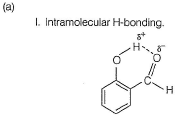I. Intermolecular H-bonding makes boiling point higher than that of I. Thus, I is more volatile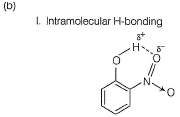II. Ortho nitrophenol is more volatile than para nitrophenol because O-Nitrophenol has intramolecular hydrogen bonding whereas para nitrophenol has inter molecular H bonding and so boils relatively at higher temperature
(c) BP of H2O > > H2S
(d) BP of CH3CH2OH (due to H-bonding) > > CH3— O — CH3

*Multiple options can be correct
QUESTION: 14

Hydrogen bonding plays a central role in the following phenomenon

Solution:

(a) When liquid water changes to ice, there is an air gap inside the cage formed due to intermolecular H-bonding. Thus, volume of ice > volume of water.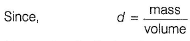Hence, density (ice) < density (water).
Thus, ice floats on water - hydrogen bonding plays its role.
(b) In gaseous phase, basicity of 1° < 2° < 3° amines due to increase of electron density at N-atomBut in aqueous (H2O) solvent, RNH2 is stabilised by solvation. Thus 1° amine is more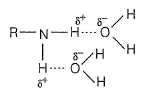basic than 3° amine (3° < 1° < 2°) amines.
(c) l+ (positive inductive effect) explains this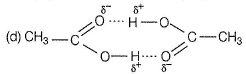Dimer due to intermolecular H- bonding.

*Multiple options can be correct
QUESTION: 15

Which of the following molecules are stabilised by intramolecular H-bonding?

Solution:

(a) intramolecular H-bonding.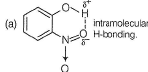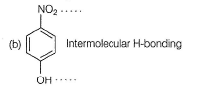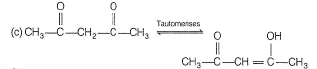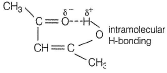*Multiple options can be correct
QUESTION: 16

Out of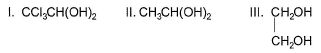Q. unstable compound is/are

Solution:

I. CCI3CH (OH)2 with two — OH groups on same carbon atom is stable due to intramolecular H-bonding.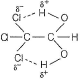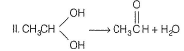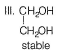QUESTION: 17

Direction (Q. Nos. 17) Choice the correct combination of elements and column I and coloumn II  are given as option (a), (b), (c) and (d), out of which ONE option is correct.

Q. Match the species in Column I with rank order of their boiling point in Column II. (Species with lowest boiling points is at SN1).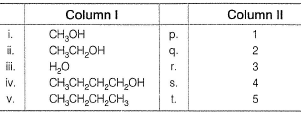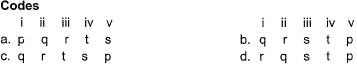Solution:

n-butane lacks H-bonding. Hence, its boiling point is least.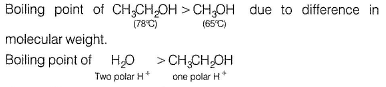due to difference in polar H+ for H-bonding.
Boiling point of CH3CH2CH2CH2OH > H2O due to much larger size of alcohol.